# 最短路问题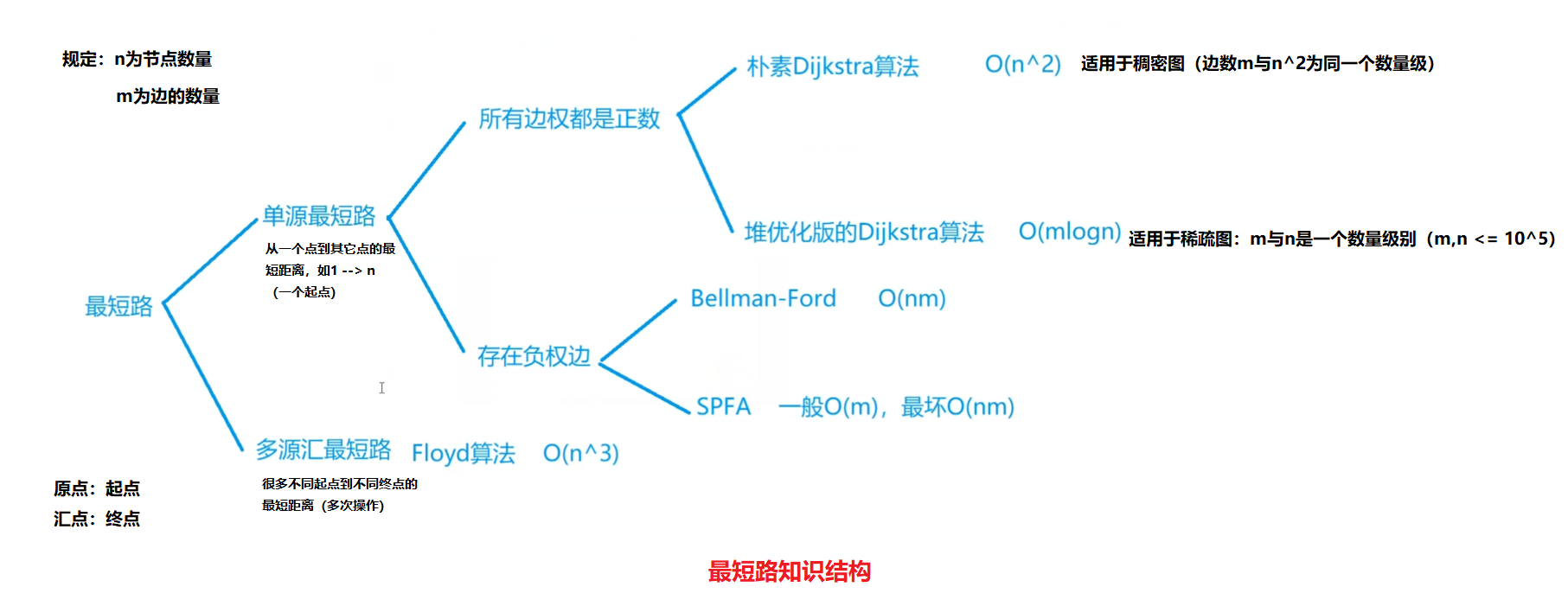## 一、单源最短路

### 1.所有边权为证数

#### （1）Dijkstra算法（朴素）

Dijkstra算法

1. 初始时，S中为空。设u是G的某一个顶点（从起点开始），把从源到u且中间只经过S中顶点的路称为从源到u的特殊路径，并用数组dist记录当前每个顶点所对应的最短特殊路径长度。
2. 每次从V-S中取出具有最短特殊路径长度的顶点u，将u添加到S中，同时对数组dist作必要的修改。
3. 一旦S包含了所有V中顶点，dist就记录了从源到所有其它顶点之间的最短路径长度。

Dijkstra算法迭代过程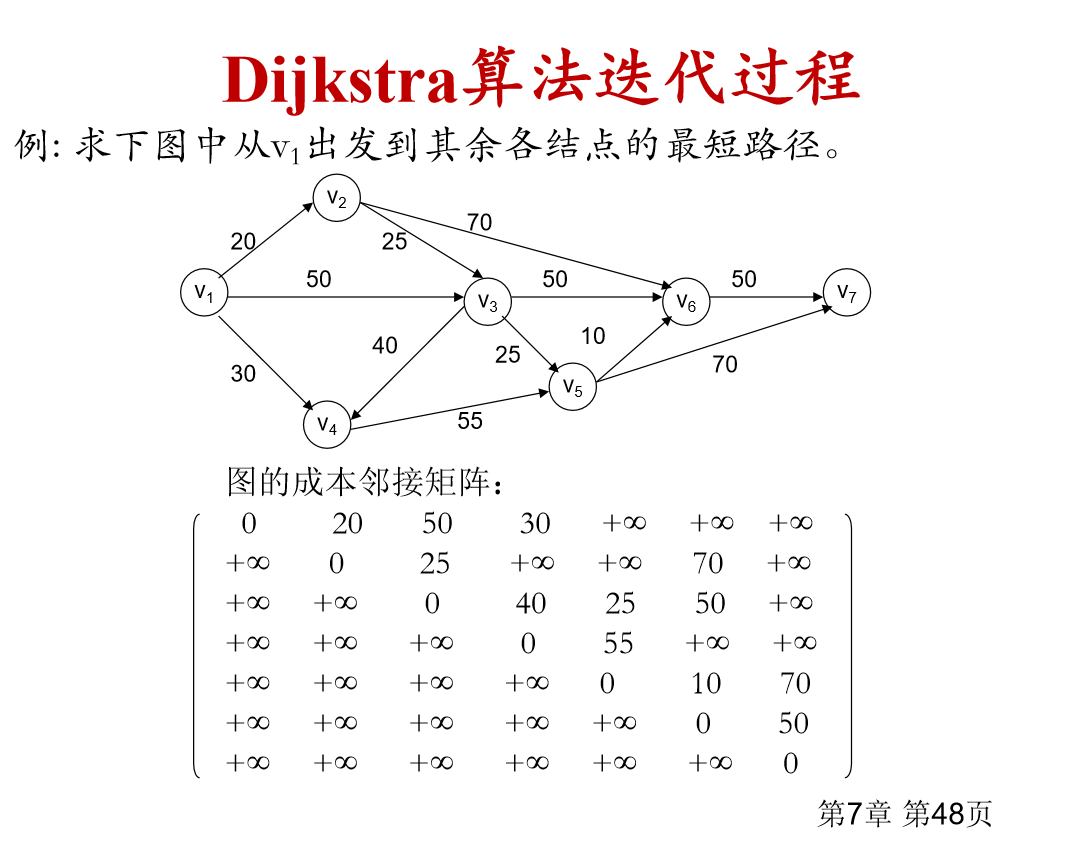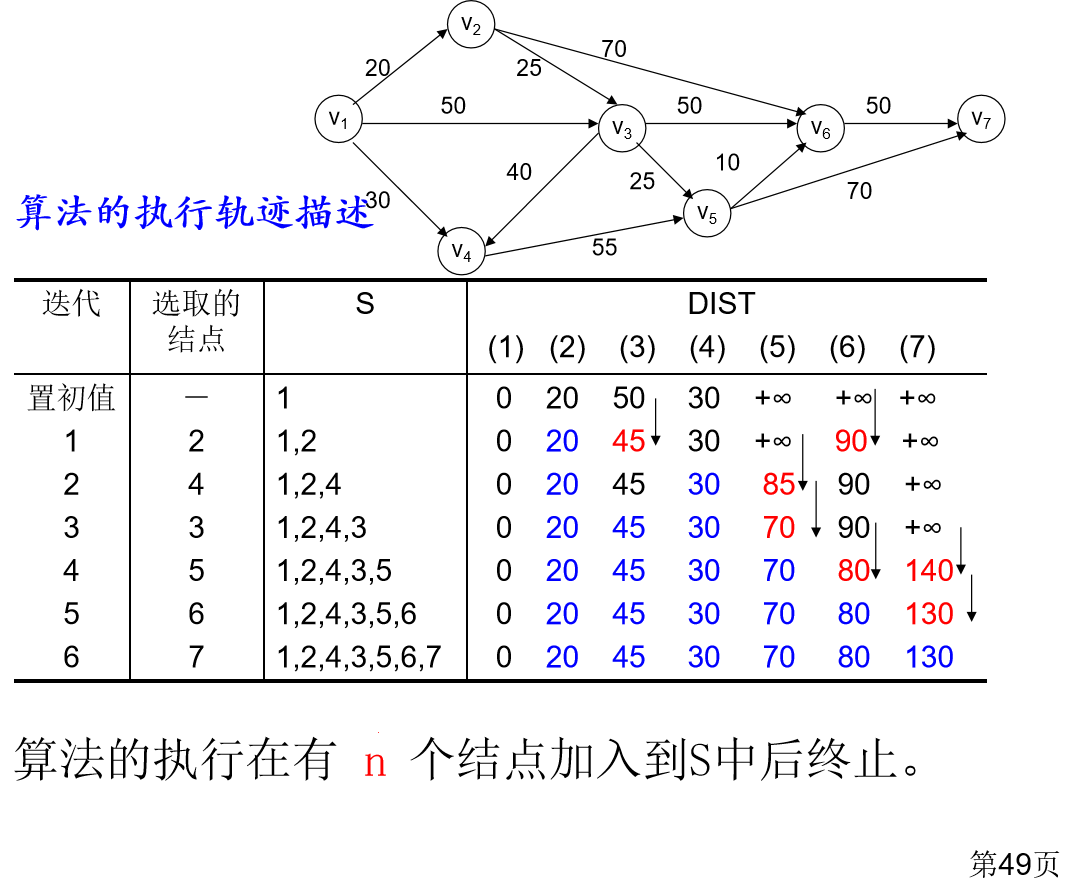【题目描述】

1≤n≤500,
1≤m≤105,

3 3
1 2 2
2 3 1
1 3 4


3


1. 初始化距离——dist数组

dist = 0,dist[i] = +无穷

2. 循环迭代过程：n次（源点也计算）。S{ }集合：记录所有当前已经确定最短距离的点！

1. t <—— 找到不在S中的，且距离最近的点（跳板）
2. t 加入S
3. t来更新其它点的距离

【参考代码】

#include <iostream>
#include <cstring>
#include <algorithm>

using namespace std;

const int N = 510;

int n, m;
int g[N][N]; //稠密图一般使用邻接矩阵
int dist[N]; //记录每个节点距离起点的最短距离距离
bool st[N]; //true表示已经确定最短路 属于s集合

int dijkstra()
{
memset(dist, 0x3f, sizeof dist); // 初始化起点到各个点的距离
dist = 0; // 源点到源点的距离为0

// 迭代循环n次, 每次可以确定一个点到源点的最短路（t点），然后更新其它点的距离
for(int i = 0; i < n; i ++)
{
int t = -1; // 用于找到第一个点，便于更新第一个点
for (int j = 1; j <= n; j ++ ) // 遍历dist数组——找到没有确定最短路径的节点中距离源点最近的点t（找到跳板）
if(!st[j] && (t == -1 || dist[t] > dist[j])) // 不在S中的，且距离最近的点
t = j;
st[t] = true; // 加入s集合

// 找到了距离最小的点t，并用最小的点t去更新其他的点到起点的距离
for (int j = 1; j <= n; j ++ ) // 更新其它点的距离（最短距离）
dist[j] = min(dist[j], dist[t] + g[t][j]);
}

if(dist[n] == 0x3f3f3f3f) return -1;
else return dist[n];
}

int main()
{
scanf("%d%d", &n, &m);

memset(g, 0x3f, sizeof g);

while (m -- )
{
int a, b, c;
scanf("%d%d%d", &a, &b, &c);
g[a][b] = min(g[a][b], c); // 如有重边：取最短边
}
int t =  dijkstra();
printf("%d", t);

return 0;
}


for(int i=0;i<n;i++) { t=-1 } 这里为什么t要赋值为 -1?

#### （2）堆优化版Dijkstra算法

1. 一号点的距离初始化为零，其他点初始化成无穷大。
2. 将一号点放入堆中。
3. 不断循环，直到堆空。每一次循环中执行的操作为：
弹出堆顶（与朴素版diijkstra找到S外距离最短的点相同，并标记该点的最短路径已经确定）。
用该点更新临界点的距离，若更新成功就加入到堆中。

【acwing 850. Dijkstra求最短路 II】

1≤n,m≤1.5×105,

3 3
1 2 2
2 3 1
1 3 4


3


【参考代码】

#include <iostream>
#include <cstring>
#include <algorithm>
#include <queue>

using namespace std;

//first:该节点到起点的距离 second:节点编号
typedef pair<int, int> PII;

const int N = 1e6 + 10, INF = 0x3f3f3f3f;
int h[N], w[N], e[N], ne[N], idx;
int dist[N];//存放点到源点的距离
bool st[N];
int n, m;

void add(int a, int b, int c)  // 添加一条边a->b，边权为c
{
e[idx] = b, w[idx] = c, ne[idx] = h[a], h[a] = idx ++;
}

int dijkstra()
{
//1.
memset(dist, 0x3f, sizeof dist);//初始化距离
priority_queue<PII, vector<PII>, greater<PII> > heap;//用堆来维护最短距离
//小根堆的定义方式,PII的第一个变量存储的是距离,第二个变量存储的是该点的编号,内部按照第一个变量排序,即按距离排序

dist = 0;
heap.push({0, 1});// 1号点已知道距离，加进来

//2.
while(heap.size())
{
//拿到不在集合中 且距离最近的点t （拿到跳板t）
auto t=heap.top();//选择最小的距离的点
heap.pop();//利用完该点之后要弹出

int ver = t.second, distance = t.first;// ver表示该点的编号

if(st[ver]) continue;// 如果被访问过了(冗余备份)，跳过
st[ver] = true;

/*
堆优化版的是将距离直接加入到堆中.
例如:dist=9(在堆中{9,5},第一次更新时加入),dist=7(在堆中{7，5},第二次更新时加入)
使用时用的是dist=7,将该点({7,5})弹出后,在下一次循环中,如果{9,5}在堆顶的话,使用时
两者间肯定要选距离要小的那个,不能使用{9,5}重复更新,所以要用st数组进行标记
*/

for(int i = h[ver]; i != -1; i =ne[i])
{
int j = e[i];
if(dist[j] > dist[ver] + w[i])//如果j到起点的距离大于ver到1的距离加上ver到j的距离，就更新值的大小
{
dist[j] = dist[ver] + w[i];
heap.push({dist[j], j}); // 最新的跳板t加入堆

/*
更新距离之后将该点的距离加入到堆中,这也是上述为何要进行标记的原因,
因为一个点的距离加入堆的次数可能有两次甚至更多,这样会影响到其他的点
例如:
{9,5},{7,5},{10,6},如果{7,5}被弹出后,堆中剩余的是{9,5},{10,6},堆顶
的元素是{9,5}而5这个点的距离已经被使用过了,所以要将{9,5}这个点忽视掉
*/
}
}
}

if(dist[n] == INF) return -1;
else return dist[n];
}

int main()
{
cin >> n >> m;
memset(h, -1, sizeof h);

while (m -- )
{
int a, b, c;
cin >> a >> b >> c;
}

int t = dijkstra();
cout << t;

return 0;
}


### 2.存在负权边

#### （1）Bellman-Ford

Bellman - ford 算法是求含负权图的单源最短路径的一种算法，效率较低，代码难度较小。其原理为连续进行松弛，在每次松弛时把每条边都更新一下，若在 n-1 次松弛后还能更新，则说明图中有负环，因此无法得出结果，否则就完成。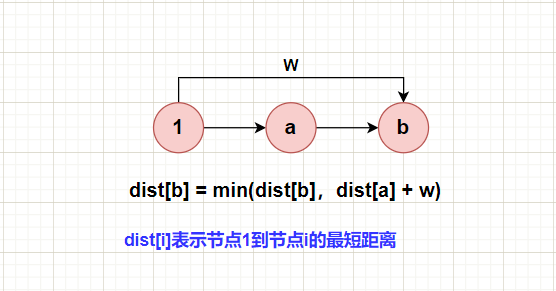for n 次
{
备份数据，防止串联

for 所有边 a ---> b (w)
{
//更新（松弛操作）
dist[b] = min(dist[b], backup[a] + w);
}
}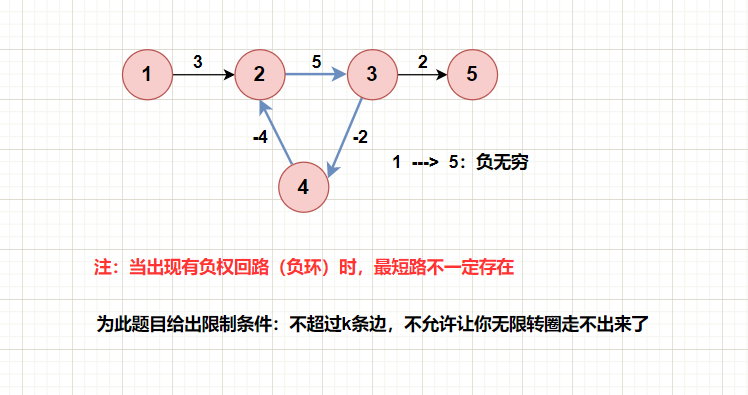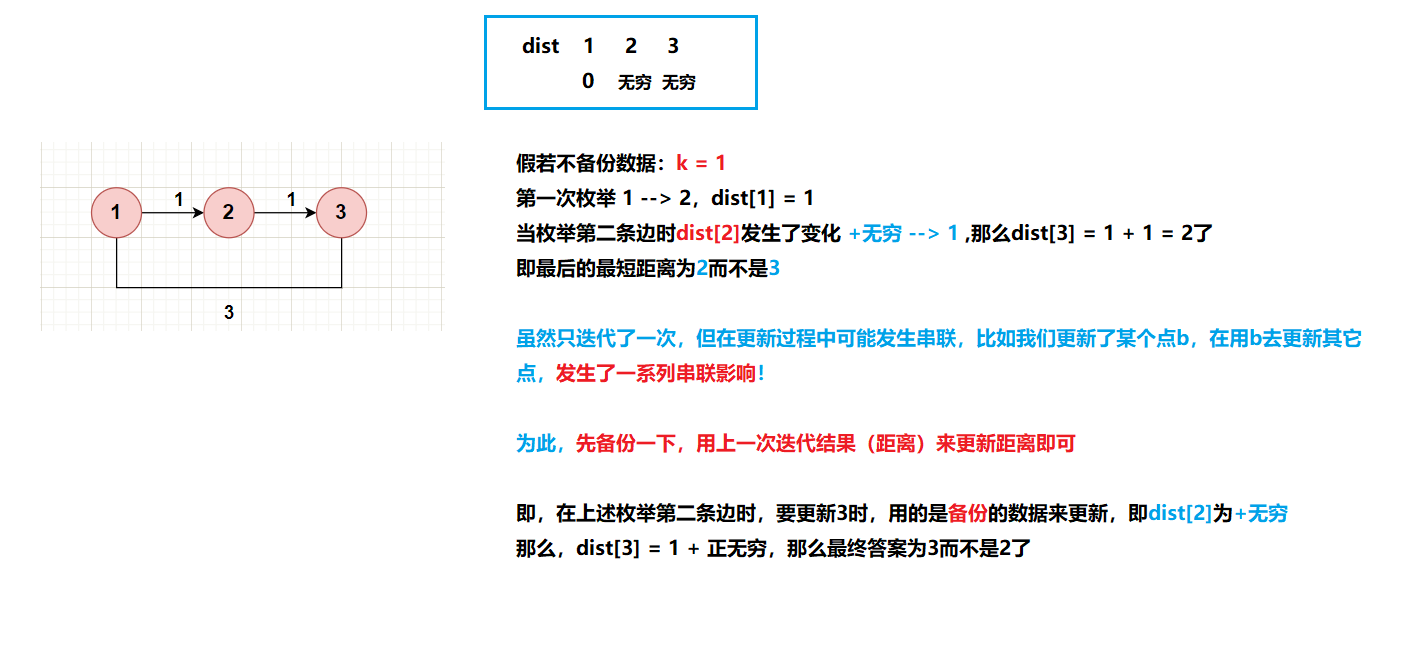5号节点距离起点的距离是无穷大，利用5号节点更新n号节点距离起点的距离，将得到109−2，109−2, 虽然小于10^9, 但并不存在最短路，(在边数限制在k条的条件下)。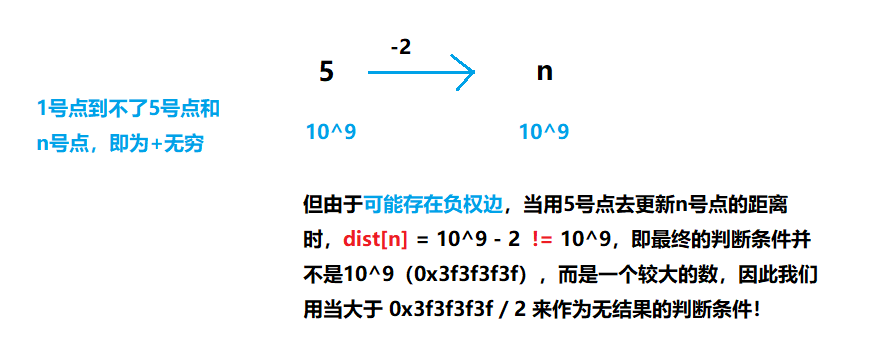1≤n,k≤500,
1≤m≤10000,

3 3 1
1 2 1
2 3 1
1 3 3


3


【参考代码】

#include <iostream>
#include <cstring>
#include <algorithm>

using namespace std;

const int N = 510, M = 1e5 + 10;

int dist[N];//dist[i]表示节点1到节点i的最短距离
int back[N];//备份数组防止串联
int n, m, k;//k代表最短路径最多包涵k条边

struct Edge
{
int a, b, w;

}edges[M];//结构体存边

int bellman_ford()
{
//初始化距离数组
memset(dist, 0x3f, sizeof dist);
dist = 0;

//1.迭代k次（不超过k条边）
for(int i = 0; i < k; i ++)
{
memcpy(backup, dist, sizeof dist);// 备份
for (int j = 0; j < m; j ++ )//遍历枚举所有条边
{
int a = edges[j].a, b = edges[j].b, w = edges[j].w;
//更新距离：三角不等式
dist[b] = min(dist[b], backup[a] + w);//每次用备份的距离（上一次）更新新距离，避免发生串联

}
}
}

int main()
{
cin >> n >> m >> k;
for (int i = 0; i < m; i ++ )
{
int a, b, w;
cin >> a >> b >> w;
edges[i] = {a, b, w};
}

int t = bellman_ford();
if(dist[n] > 0x3f3f3f3f / 2) puts("impossible");
else cout << dist[n];

return 0;
}


#### （2）SPFA

$SPFA 算法是 Bellman-Ford算法 的队列优化算法的别称，通常用于求含负权边的单源最短路径，以及判负权环。SPFA一般情况复杂度是O(m) 最坏情况下复杂度和朴素 Bellman-Ford 相同，为O(nm)。$

Bellman_ford算法会遍历所有的边，但是有很多的边遍历了其实没有什么意义，我们只用遍历那些到源点距离变小的点所连接的边即可，只有当一个点的前驱结点更新了，该节点才会得到更新；因此考虑到这一点，我们将创建一个队列每一次加入距离被更新的结点。

dist[b] = min(dist[b], dist[a] + w)只有当dist[a]小了dist[b]才会变小，因此每次迭代操作队列里边存储的是所有变小的a(节点)，用它来更新后面与它相连的所有点（更新它的所有后继），这些点可以理解为【待更新其它点的点】。（用更新过的点去更新其它点，只有我变小了，我后面的人也才会跟着变小！）

	st[N]; // 判断某点是否被使用过

1. queue <--- 1

2.while(队列不空)
{
//2.1
t <--- q.front();
q.pop();
//2.2枚举t的所有出边 更新t的所有出边 t ---> b (w)
{
更新
判重，新点加入队列 queue <--- b
}

}
3.队空 结束


st数组的作用：判断当前的点是否已经加入到队列当中了；已经加入队列的结点就不需要反复的把该点加入到队列中了，就算此次还是会更新到源点的距离，那只用更新一下数值而不用加入到队列当中。

【spfa求最短路】

1≤n,m≤105,

3 3
1 2 5
2 3 -3
1 3 4


2


【参考代码】

#include <iostream>
#include <cstring>
#include <algorithm>
#include <queue>

using namespace std;

const int N = 1e6 + 10, INF = 0x3f3f3f3f;

int h[N], w[N], e[N], ne[N], idx;
int dist[N];//存放点到源点的距离
bool st[N];
int n, m;

void add(int a, int b, int c)  // 添加一条边a->b，边权为c
{
e[idx] = b, w[idx] = c, ne[idx] = h[a], h[a] = idx ++;
}

int spfa()
{
memset(dist, 0x3f, sizeof dist);
dist = 0;

queue<int> q;
q.push(1);
st = true;

while(q.size())
{
int t = q.front();
q.pop();
st[t] = false;//取出队列后 标记为已用过

//用t去去更新所有出边（后继）
for(int i = h[t]; i != -1; i = ne[i])
{
int j = e[i];
if(dist[j] > dist[t] + w[i])
{
dist[j] = dist[t] + w[i];
if(!st[j])//如果j节点尚未加入队列（判重）
{
q.push(j);
st[j] = true;//待更新其它点的点入队 标记
}
}
}
}

return dist[n];
}

int main()
{
cin >> n >> m;
memset(h, -1, sizeof h);

while (m -- )
{
int a, b, c;
cin >> a >> b >> c;
}

int t = spfa();
if(t == INF) puts("impossible");
else cout << t;

return 0;
}


【spfa判断负环】

1≤n≤2000,
1≤m≤10000,

3 3
1 2 -1
2 3 4
3 1 -4


Yes


1、dist[x] 记录虚拟源点x的最短距离

2、cnt[x] 记录当前x点到虚拟源点最短路的边数，初始每个点到虚拟源点的距离为0，只要他能再走n步，即cnt[x] >= n，则表示该图中一定存在负环，由于从虚拟源点到x至少经过n条边时，则说明图中至少有n + 1个点，表示一定有点是重复使用

3、若dist[j] > dist[t] + w[i],则表示从t点走到j点能够让权值变少，因此进行对该点j进行更新，并且对应cnt[j] = cnt[t] + 1,往前走一步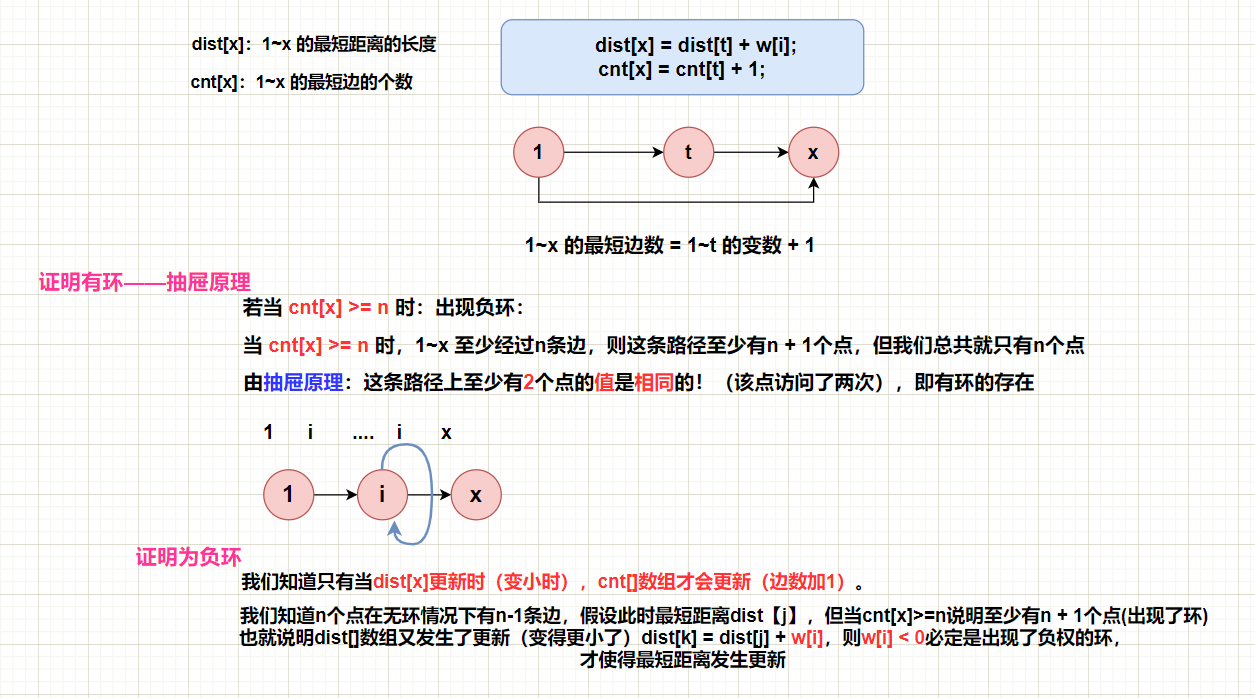【注意】：该题是判断是否存在负环，并非判断是否存在从1开始的负环，因此需要将所有的点都加入队列中，更新周围的点

【参考代码】

#include <iostream>
#include <cstring>
#include <algorithm>
#include <queue>

using namespace std;

const int N = 1e6 + 10, INF = 0x3f3f3f3f;

int h[N], w[N], e[N], ne[N], idx;
int dist[N];//存放点到源点的距离
int cnt[N];//cnt[x] 表示 当前从1-x的最短路的边数
bool st[N];
int n, m;

void add(int a, int b, int c)  // 添加一条边a->b，边权为c
{
e[idx] = b, w[idx] = c, ne[idx] = h[a], h[a] = idx ++;
}

bool spfa()
{

// 这里不需要初始化dist数组为 正无穷/初始化的原因是， 如果存在负环， 那么dist不管初始化为多少， 都会被更新
queue<int> q;

//不仅仅是1了， 因为点1可能到不了有负环的点， 因此把所有点都加入队列
for(int i = 1; i <= n; i ++)
{
q.push(i);
st[i] = true;
}

while(q.size())
{
int t = q.front();
q.pop();
st[t] = false;//取出队列后 标记为已用过

//用t去去更新所有出边（后继）
for(int i = h[t]; i != -1; i = ne[i])
{
int j = e[i];
if(dist[j] > dist[t] + w[i])
{
dist[j] = dist[t] + w[i];
cnt[j] = cnt[t] + 1;//维护cnt数组
if(cnt[j] >= n) return true;

if(!st[j])
{
q.push(j);
st[j] = true;
}
}

}
}
return false;
}

int main()
{
cin >> n >> m;
memset(h, -1, sizeof h);

while (m -- )
{
int a, b, c;
cin >> a >> b >> c;
}

if(spfa()) puts("Yes");
else puts("No");

return 0;
}


## 二、多源最短路

### Floyd

void floyd()
{
for(int k = 1; k <= n; k ++)
for(int i = 1; i <= n; i ++)
for(int j = 1; j <= n; j ++)
dist[i][j] = min(dist[i][j], dist[i][k] + dist[k][j]);
}


1≤n≤200,
1≤k≤n2
1≤m≤20000,

3 3 2
1 2 1
2 3 2
1 3 1
2 1
1 3


impossible
1


【参考代码】

• f[i, j, k]表示从i走到j的路径上除i和j点外只经过1到k的点的所有路径的最短距离。那么f[i, j, k] = min(f[i, j, k - 1), f[i, k, k - 1] + f[k, j, k - 1]
因此在计算第k层的f[i, j]的时候必须先将第k - 1层的所有状态计算出来，所以需要把k放在最外层。

• 读入邻接矩阵，将次通过动态规划装换成从i到j的最短距离矩阵

• 在下面代码中，判断从ab是否是无穷大距离时，需要进行if(t > INF/2)判断，而并非是if(t == INF)判断，原因是INF是一个确定的值，并非真正的无穷大，会随着其他数值而受到影响，t大于某个与INF相同数量级的数即可

#include <iostream>
#include <cstring>
#include <algorithm>

using namespace std;

const int N = 210, INF = 1e9;

int dist[N][N]; // 存储 x 到 y 的最短距离

int n, m, Q;

void floyd()
{
for(int k = 1; k <= n; k ++)
for(int i = 1; i <= n; i ++)
for(int j = 1; j <= n; j ++)
dist[i][j] = min(dist[i][j], dist[i][k] + dist[k][j]);
}

int main()
{
cin >> n >> m >> Q;

// 初始化
for (int i = 1; i <= n; i ++ )
for (int j = 1; j <= n; j ++ )
if(i == j) dist[i][j] = 0;
else dist[i][j] = INF;

// 读入
while (m -- )
{
int a, b, c;
cin >> a >> b >> c;
dist[a][b] = min(dist[a][b], c); // 避免了重边和自环，只会保存最小的距离
}

floyd();

// 查询
while(Q --)
{
int a, b;
cin >> a >> b;

int t = dist[a][b];
if(t > INF / 2) puts("impossible");
else cout << t << endl;
}

return 0;
}


## 三、总结

acwing算法基础课posted @ 2022-01-14 11:45  时间最考验人  阅读(205)  评论(0编辑  收藏  举报